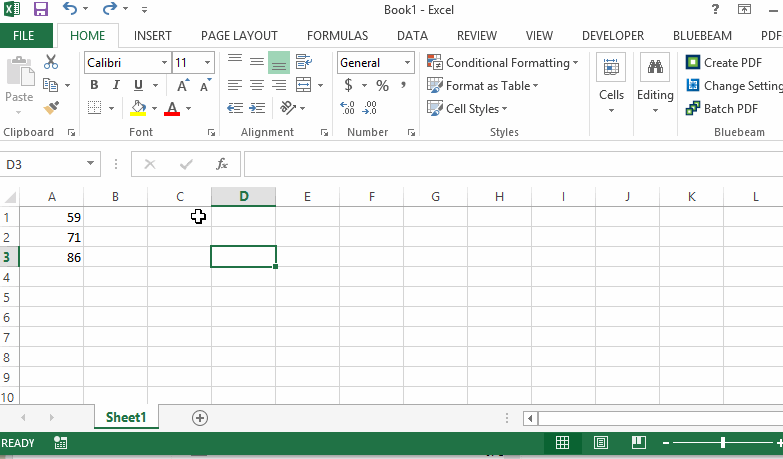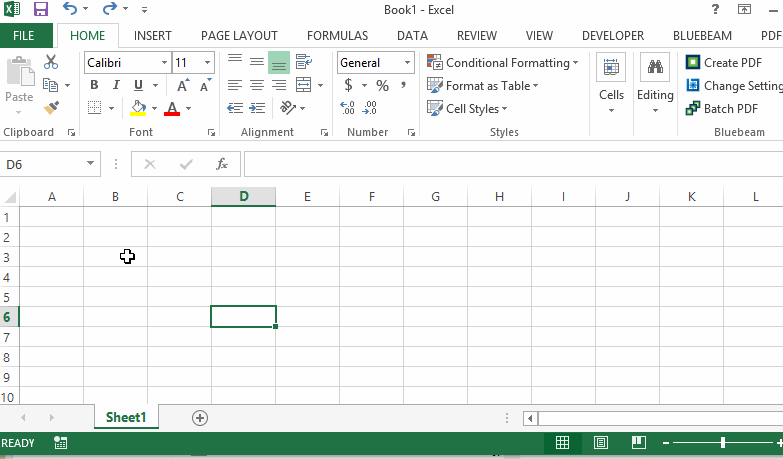# How to Multiply in Excel

One of the most basic question to start learning Microsoft Excel is to how to add cells. This tutorial will demonstrate how to sum values within multiple cells.

## 1. Multiply using cell reference

Click a cell where you want to show the result, then type in = sign from your keyboard. Select each cells you want to multiply separated by * sign from the keyboard and press Enter to return the product. See screen recording below for details.## 2. Multiply using in-cell values

Start by typing = and then type in the numbers to multiply separated by * symbol. Press Enter to return the product of these values. See screen recording below for details.Do you know that Microsoft Excel are also capable of solving fractions? Learn how to multiply fractions in Excel now!